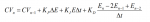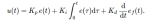# Derivative Filter in velocity-form PID algorithm?

#### mark2784

Hi everyone!

I have wondered about implementation a derivative filter in velocity form of PID algorithm (for example exponential moving everage filter). I haven't seen in any books or forum about necessity to use derivative filter in velocity algorithm.

However, I think derivative filter is necessary in such form as below (source:https://twcontrols.com/):Thank you very much for help!

•Carl

#### pnachtwey

I am not home or at work at the moment so I can't give you the exact answer but if I give you glues you may be able to figure it out by yourself.
First. The form of the PID will be that of a digital filter like
"y=A1*y(n-1)+A2*y(n-2)+B0*x+B1*x(n-1)+B2*x(n-2)"
What is wrong with this site? Why can I type "y"
Without quotes? A real engineering site would use Mathjax and support LaTeX.

Ys are outputs or control outputs. Xs are inputs or errors.
I would add min and max functions to limit the values of Y
You can see this is very efficient as there are only 5 multiply and adds.
The problem is computing A1,A2,B0,B1,B2. This gets messy but I have it done. I am just not where I can access it.
To generate the 5 coefficients, I started with the Laplace transform of a PID with a low pass filter on the derivative.
Normally a PID is expressed like this:
PID(s)=Ki/s+Kp+Kd*s
but you want to add a low pass filter p/(s+p) to the derivative term so you get
PID(s)=Ki/s+Kp+Kd*s*(p/(s+p))
p is the cutoff frequency of the low pass filter, pole, in radians/second.
Next you use the Tustin's approximation for s. s=(2/T)*((1-z)/(1+z)) for all occurrences s.
Now the formula is in the z domain ( discrete ) and it is messy and needs to be simplified.
The coefficients of z are the values for the 5 coefficients above. The highest power of z in the denominator will usually not be one but it must be forced to be one by dividing ALL the coefficients by the coefficient of the highest power of z in the denominator. The math is messy but the results are very efficient.

This is a good topic and there isn't much info on how to approach these kinds of problem.
I have a YouTube channel. Peter Ponders PID. I will make a video about this but it will have to wait until I get home and have time.

Other good topics would be converting the PID to the z ( discrete ) domain using matched z transforms.

#### mark2784

I have derive PID algorithm in velocity form with filtering from equation in time domain. It was quite simple, because I have read about derivation of velocity form, but without derivative form.

However, I am not sure, if due to the components of velocity form algorithm, the form don't have filtering properties without implementing other solutions.

#### pnachtwey

I made a YouTube video that covers what must be done to add a low pass filter to an incremental ( velocity ) form of PID here.

#### mark2784

I made a YouTube video that covers what must be done to add a low pass filter to an incremental ( velocity ) form of PID here.
Thank you very much!
What do you think about derivation velocity form with Low Pass filter on differentiator based on time domain equation?I have derived such algorithm based on backward difference, but a e_f(k) is output from LP filter of e(k):
e_f(k) = (1-a)*e_f(k-1) + a*e(k)

#### pnachtwey

View attachment 2164
Some of my familiars claim that due to expression (E- 2*E(n-1) + E(n-2)) it is not necessary to apply derivative filter for PV value.
To me, it is necessary.
I agree.
In your previous post, if e_f(k) is done properly, the result should be the same as mine. One way to test is using a simulation like I did.

What people don't realize is that much of the "noise" you are trying to filter out is due to quantizing due to coarse feed back resolution. The other problem is sample jitter where the feed back is not sampled at exactly constant intervals. This makes it difficult to calculate a rate of change of the PV or actual position in motion control. In motion control I often need a second derivative gain that is a gain applied to the error between the target and actual acceleration. The acceleration calculation is subject to feed back resolution quantizing. There are three solutions. 1) finer resolution feed back. This option may not be available. 2) use a low pass filter on the output from the PID. 3) Use a Luenberg observer. The Luenberg observer requires an accurate model. I obtain the model using system identification. The advantage of the observer is that the estimated position, velocity and acceleration is often more accurate than the measured position, velocity and acceleration. The estimated values have almost infinite resolution because the model is calculated using floating point. I have a videos on system identification and observers in my Peter Ponder's PID YouTube channel

•mark2784

#### mark2784

I agree.
In your previous post, if e_f(k) is done properly, the result should be the same as mine. One way to test is using a simulation like I did.

What people don't realize is that much of the "noise" you are trying to filter out is due to quantizing due to coarse feed back resolution. The other problem is sample jitter where the feed back is not sampled at exactly constant intervals. This makes it difficult to calculate a rate of change of the PV or actual position in motion control. In motion control I often need a second derivative gain that is a gain applied to the error between the target and actual acceleration. The acceleration calculation is subject to feed back resolution quantizing. There are three solutions. 1) finer resolution feed back. This option may not be available. 2) use a low pass filter on the output from the PID. 3) Use a Luenberg observer. The Luenberg observer requires an accurate model. I obtain the model using system identification. The advantage of the observer is that the estimated position, velocity and acceleration is often more accurate than the measured position, velocity and acceleration. The estimated values have almost infinite resolution because the model is calculated using floating point. I have a videos on system identification and observers in my Peter Ponder's PID YouTube channel

Sorry for excursion, but what do you think about PI-like fuzzy logic controller with change of output as one of input signal instead of classical change of deviation? Do you think if such solution could improve perfomance of system?

#### pnachtwey

I don't think much of fuzzy logic. It is an attempt to control a system without knowing anything about it and the results are at best, FUZZY. This video will give you an idea of what I really think about FL
Most people that push FL don't know anything about system identification and pole placement. People say that FL can compensate for non-linear systems. It can sort of with a lot of trial and error. With a model one can change the PID gains on-the-fly as a function of the state.

This is a video I made in response to a student that needed to control the water level in a system were a pump pumped water into tank #1.

•mark2784

#### mark2784

I don't think much of fuzzy logic. It is an attempt to control a system without knowing anything about it and the results are at best, FUZZY. This video will give you an idea of what I really think about FL
Most people that push FL don't know anything about system identification and pole placement. People say that FL can compensate for non-linear systems. It can sort of with a lot of trial and error. With a model one can change the PID gains on-the-fly as a function of the state.

This is a video I made in response to a student that needed to control the water level in a system were a pump pumped water into tank #1.

I have posted thread about sampling time for Kalman Filters and controller. It's a rule of thumb that controller should have bandwith 5x lower than measurement. It is correct to set the same sampling time for Kalman Filter and decrease bandwith of controller by lowering the gain? Or is it necessary to increase sampling time of controller to 5x times greater than Kalman filter?

#### pnachtwey

I don't understand. The gains of the Kalman filter require knowing the values of the process noise and the measurement noise to find a ratio the effective weights, K gains, between the two. Were you select the band width?

An Alpha Beta Gamma filter computes the gain by choosing a frequency. A good place to start is square root of the sample frequency * the frequency of motion. So if the same frequency is 1000 Hz and the frequency of motion is 10 Hz set the Alpha-Beta-Gamma filter to 100 Hz. This way it will reduce the sampling noise but not interfere with the motion.

#### mark2784

I don't understand. The gains of the Kalman filter require knowing the values of the process noise and the measurement noise to find a ratio the effective weights, K gains, between the two. Were you select the band width?

An Alpha Beta Gamma filter computes the gain by choosing a frequency. A good place to start is square root of the sample frequency * the frequency of motion. So if the same frequency is 1000 Hz and the frequency of motion is 10 Hz set the Alpha-Beta-Gamma filter to 100 Hz. This way it will reduce the sampling noise but not interfere with the motion.
I have wondered about selecting proper sampling time for executing Kalman Filter algorithm for speed estimation and sampling time for speed controller. I have read in some books that for example in cascaded position control system, position controller should have bandwith at least 5 times slower than bandwith of speed controller. As I understand bandwith of controller depends on from controller gains, so is it necessary to set 5 times longer sampling time for position controller or is it enough to set lower gain for example of P controller?

I have also read in a forum that similar relationship regards control system with Kalman filter estimate in feedback - estimate should be at least 5 times faster than controller. Could you resolve my doubts?